# Two Way Slab Design by Direct Design Method as per ACI 318-11Reading time: 1 minute

Two-way slab design by direct design method as per ACI 318-11, step by step procedure and limitations of a direct design method for the two-way slab is presented. Generally, there are two types of slabs which are one-way and two-way slabs. The one-way slab is deflected in a one-way direction and primary reinforcement is placed in one direction whereas the two-way slab deflects in two directions and primary reinforcement placement is in two directions. ACI 318-11 Code provides two methods for two-way slab design one of which is the direct design method. The direct design method, which could have been named the direct analysis method because it determines or prescribes moments for different parts of the slab panel without the need to conduct structural analysis, is explained in the following sections.

## Procedures of Two Way Slab Design by Direct Design Method

1. Determine slab type and layout
2. Choose slab thickness that should be adequate for avoiding excessive deflections and satisfy shear at the interior and exterior columns.
3. Choose design method (direct design method in this case)
4. Calculate positive and negative moments in the slab
5. Distribute moments across slab width
6. Specify portion of moments to the beams, if beams are present
7. Compute reinforcements for moments that found in two previous points
8. Check shear strength

## Limitations of Direct Design Method

• There must be at least three continuous spans in each direction. If there are fewer panels, the interior negative moments tend to be too small.
• Panels should be rectangular and the ratio of longer/ shorter spans within the panel must not exceed 2 otherwise one-way actions will prevail.
• In each direction, successive span lengths must not differ by more than one-third of the largest span length.
• Column offset of more than 10% of the span (in the direction of offset) from either axis between the centerline of the successive column is not permitted.
• This method is applicable for slabs that subjected to gravity load only.
• f beams were used, beam relative stiffness between two perpendicular directions must be between 0.2-0.5.

## Two Way Slab Design by Direct Design Method

Before the start of the two-way slab design, slab depth should be determined in addition to specifying column strips and middle strips. In this article, it is assumed that slab thickness is determined but the calculation of column and middle strips is explained in the following section:

### Column and middle strips

There are continuous variations of moments across slab panels therefore to help placement of steel, design moments are averaged over column strips and middle strips. The column strips are located over columns and have a width on each side of the column centerline equal to a smaller panel dimension divided by four and middle strips are located between two column strips. Figure-1 and Figure-2 illustrate the middle strip and column strip for long and short directions of the panel.Figure-1: Column and Middle Strip in Short Direction of the PanelFigure-2: Column and Middle Strips in Long Direction of the Panel

### Total static moment for a span (Mo):

Clear span (ln) which is extended from face to face of walls or columns, in the direction of moments is used to compute total static moment in a panel. A factored moment in as span is calculated as per the following equation: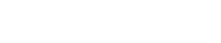Where: wu: Ultimate load per unit area of the slab ln: Clear span in (Mo) direction measured face to face of walls, columns, brackets, or capitals, and should be equal or larger than 0.65l1 l1: span length in the direction of (Mo) l2: span length perpendicular to l1 The above parameters are illustrated for both short and long direction of the slab in Figure-3Figure-3: Column and middle strip in both direction of the slab with necessary parameters for calculating total static moment

### Distribution of total static moment to positive and negative moments

#### 1. For interior spans:

Total static moments are distributed to positive and negative moments as per the following ratios: Negative factored moment -Mu = 0.65Mo Positive factored moment +Mu = 0.35Mo

#### 2. For edge spans:

The total static moment is distributed to negative exterior moment, interior moment, and negative interior moment as per Table-1.

Table-1: Factors applied to static moment for positive and negative momentsEnd span and interior span slabs are illustrated in Figure-4Figure-4: Distribution of total static moment to critical sections in interior and end spans

### Lateral distribution of moments between column and middle strips

After assigning total static moments into positive and negative moments, it is necessary to distribute these moments to middle and column strips. For design purposes, moments are assumed to be uniformly distributed to column and middle strips except beams are present. Positive and negative moment distribution to column and middle strips is depended number of parameters which will be explained in the following sections: A. The ratio of (l2/l1), B. Relative stiffness (af) of beams and slabs spanning in each direction: is expressed as: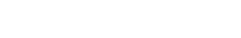Where: Ecb and Ecs: concrete modulus of elasticity of beam and slab that is usually the same Ib and Is: Moment of inertia of beam and slab respectively. af1 and af2: used to specify calculated (af) for the direction l1 and l2 respectively. Figure 5 and Figure 6 illustrate how to find a moment of inertia for edge beam, internal beam, internal and edge slabs respectively:Figure-5: Portion of slabs to be included for the moment of inertia calculation, edge beam (left side) & internal beam (right side)Figure-6: Dimensions of internal and external slab for a moment of inertia calculations

C. Degree of tensional stiffness provided by edge beams which is expressed by parameter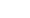: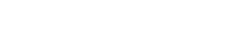Where: Is: slab moment of inertia of a slab that is spanning in the direction l1 bounded by panel centerline in the direction of l2. C: Related to tensional rigidity of the effective transverse beam and it can found by equation-4 and illustrated in Figure-7.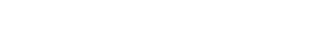Where: x and y: Smaller and larger dimension respectivelyFigure-7: Two possible subdivisions of L-section in rectangles for torsion constant C

With these parameters defined positive and negative moments are assigned to a column and middle strips. Table-2 used to specify the portion of positive and negative moments to column strip and interpolations when it is required. When beam is present along column line in the direction of l1, 85% of column strip moment is resisted by the beam if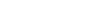and for values between zero and one interpolation should be used to the estimated amount of moment resisted by the beam. After determining column strip moments, the remained moments go to the middle strips.

Table-2: Column strip moment, percent of a total moment at the critical sectionNow, moments are assigned to different parts of slab panel, and it is easy to find reinforcement ratio for those moments.